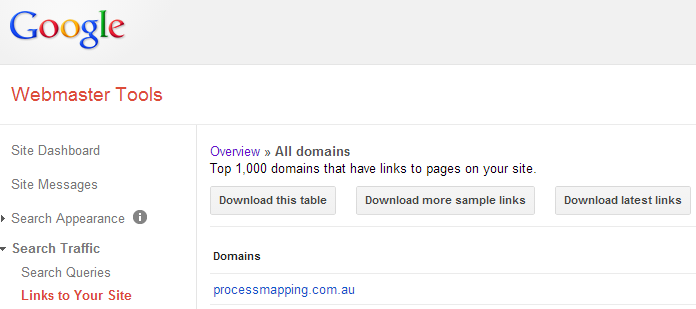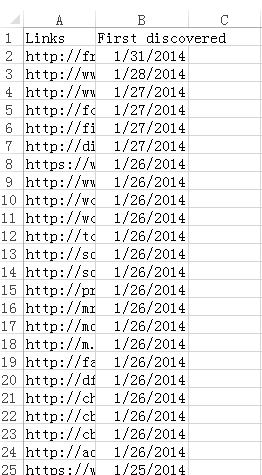×

# How to Extract Domain Names from URLs in VBA

Backlink is one of the most important factors for search engine ranking. Thus, the analysis of backlinks is significant for website owners. It is known that Google Webmaster tools can record search traffic allowing users to check the links to their sites. We can download the relevant data as figure 1.Figure 1

However, it is imperfect that the data only consists of links and dates (figure 2), which are not categorized or visualized. In my point of view, the worst part is that there is no data of domain names, which are of a higher value than the backlinks. Therefore, if we want to see the domain names, we have to process the data ourselves. Today, I would like to share my knowledge about how to extract domain names from URLs in VBA.Figure 2

# Why Programmatically Processing the Excel Data?

Some may think that it is not necessary to deal with the data in VBA. That to filter the domain names, the basic built-in functions are enough. It is true that to get the domain names, we just need to type in “=LEFT(text, FIND(“/”,text,[start_num]))”. However, this approach is not perfect. It cannot process sub domain names. For example, after executing excel functions, we can get both “a.test.com” and “b.test.com” whereas the target domain name should be “test.com”. How can we solve this complicated question? The answer is to write a program in VBA.

# How to Extract the Domain Names?

To solve the problem, we can consider the following steps:

• Create a data source of domains. E.g. “.com, .net, .org, .gov …”
``````dict = CreateObject("Scripting.Dictionary") ' use dictionary to store key & value
' initialize the data source of domains
``````
• Split “://” and “/” to get the domain names. E.g. “http://social.msdn.microsoft.com/a/b/c/d.html” -> “social.msdn.microsoft.com”
``````Dim urlArray() As String
Dim domainArrayOri() As String
Dim sourceRow, sourceCol, destinationRow, destinationCol As Integer
sourceRow = 2 ' index of source row
sourceCol = 1 ' index of source column
destinationRow = 2 ' index of destination row
destinationCol = 7 ' index of destination column

''------------------------------------------------------------------
urlArray = Split(Cells(sourceRow, sourceCol).Value, "://")
domainArrayOri = Split(urlArray(1), "/")
``````
• Split “.” to store strings in array. E.g. “social.msdn.microsoft.com” -> “social”, “msdn”, “microsoft”, “com”
``````domainArray = Split(domainArrayOri(0), ".")
``````
• Traverse the array data to find out the domain that matches the data source of domains. E.g. the domain is “com”
``````' function for checking domain
Function isDomain(tmp As String, domains() As String) As Boolean
isDomain = False
For Each domain In domains
If tmp = domain Then
isDomain = True
Exit For
End If
Next domain

End Function
``````
``````        lastIndex = UBound(domainArray, 1)
firstIndex = LBound(domainArray, 1)
count = lastIndex - firstIndex + 1
If count > 2 Then
Dim j As Integer
Dim bIsDomain As Boolean
bIsDomain = False
If lastIndex > 5 Then
lastIndex = 3
End If

For j = 2 To lastIndex
If dict.Exists(domainArray(j)) Then
' TODO:
Else
' TODO:
End If
Next j
Else
Cells(destinationRow, destinationCol).Value = domainArrayOri(0)
End If
``````
• Compose the domain names with the adjacent string “microsoft”. E.g. the final domain name is “Microsoft.com”
``````                If dict.Exists(domainArray(j)) Then
bIsDomain = True
Cells(destinationRow, destinationCol).Value = domainArray(j - 1) & "." & domainArray(j)
Else
Cells(destinationRow, destinationCol).Value = domainArrayOri(0)
End If
``````